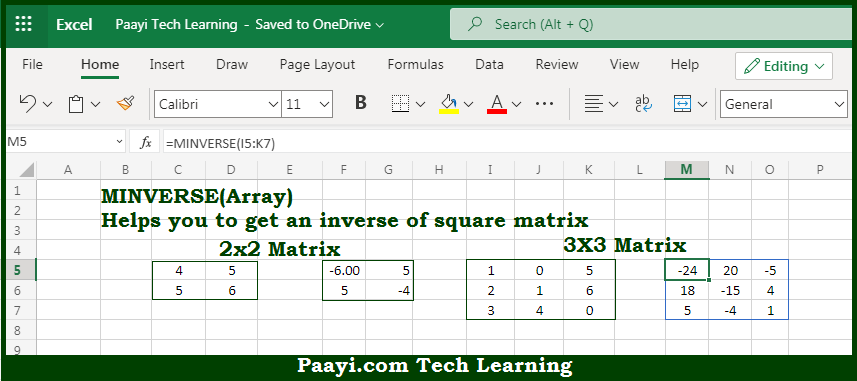# Learn How to Use Microsoft Excel MINVERSE Function

Written by | 0 Comments | 578 Views

In this article, you will learn how to use the Microsoft Excel MINVERSE function and its prime function in Microsoft Excel. You will also get to know the Microsoft Excel MINVERSE function return value and syntax with the help of some examples.

Microsoft Excel MINVERSE Function

The main function of the Microsoft Excel MINVERSE function is to get the inverse matrix as an array with the same dimensions. That implies, with the help of MINVERSE function you can able to return the inverse matrix of the given array. It should be noted that the input array must contain numbers only and should be a square matrix, with equal rows and columns. The result will be the inverse matrix with the same dimensions as the array provided. So, with the help of MINVERSE function, you can able to get the inverse matrix as an array with the same dimensions.

Return Value of MINVERSE Function

The return value will be the inverse matrix as an array in the same direction.

Syntax of MINVERSE Function

=MINVERSE(array)

Where the arguments:

• array: This is the square array of numbers only.

## How to Use Microsoft Excel MINVERSE Function?So we know that Microsoft Excel MINVERSE function you can able to get the inverse matrix as an array in the same direction. That implies, with the help of MINVERSE function you can able to return the inverse matrix of the given array. It should be noted that the input array must contain numbers only and should be a square matrix, with equal rows and columns.

The result will be the inverse matrix with the same dimensions as the array provided. So, with the help of MINVERSE function, you can able to get the inverse matrix as an array with the same dimensions.